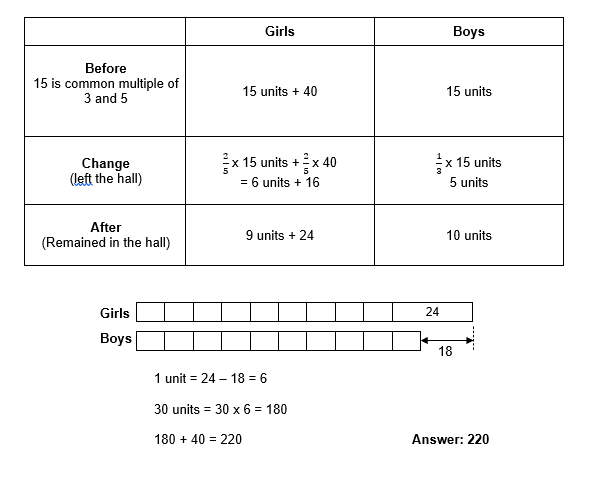# Question

There were 40 more girls than boys in the school hall. 2/5 of the girls and 1/3 of the boys left the hall. In the end, there were 18 more girls than boys remaining in the hall.

Find the total number of girls and boys in the hall at first.  Ans: 220

Source: 2020 P6 CA1 ACS(J)

I’ll let some folks with better knowledge of models to solve this in another way.

By simultaneous equations, this is my method:

Let G be the number of girls originally.
Let B be the number of boys originally.

G – B = 40
(1) G = B + 40

After people left the hall, we are left with
(3/5)G – (2/3)B = 18
=> G – (5/3)(2/3)B = (5/3)18
(2) G – (10/9)B = 30

Substituting (1) into (2),
B + 40 – (10/9)B = 30
=> -(1/9)B = -10
=> B = 90

So G = 90 + 40 = 130

So the total number of boys and girls at first is 130 + 90 = 220 #

0 Replies 2 Likes ✔Accepted Answer0 Replies 2 Likes